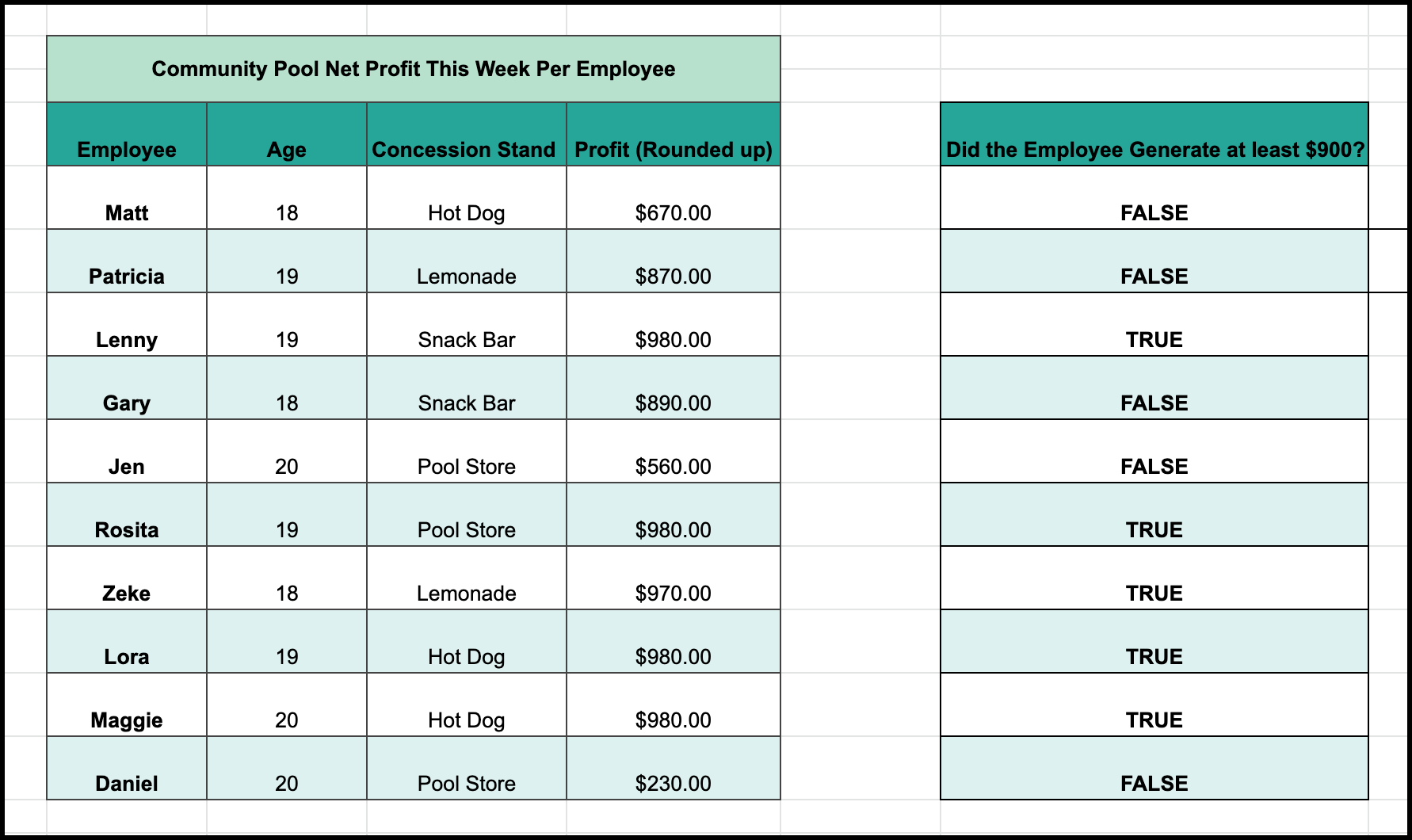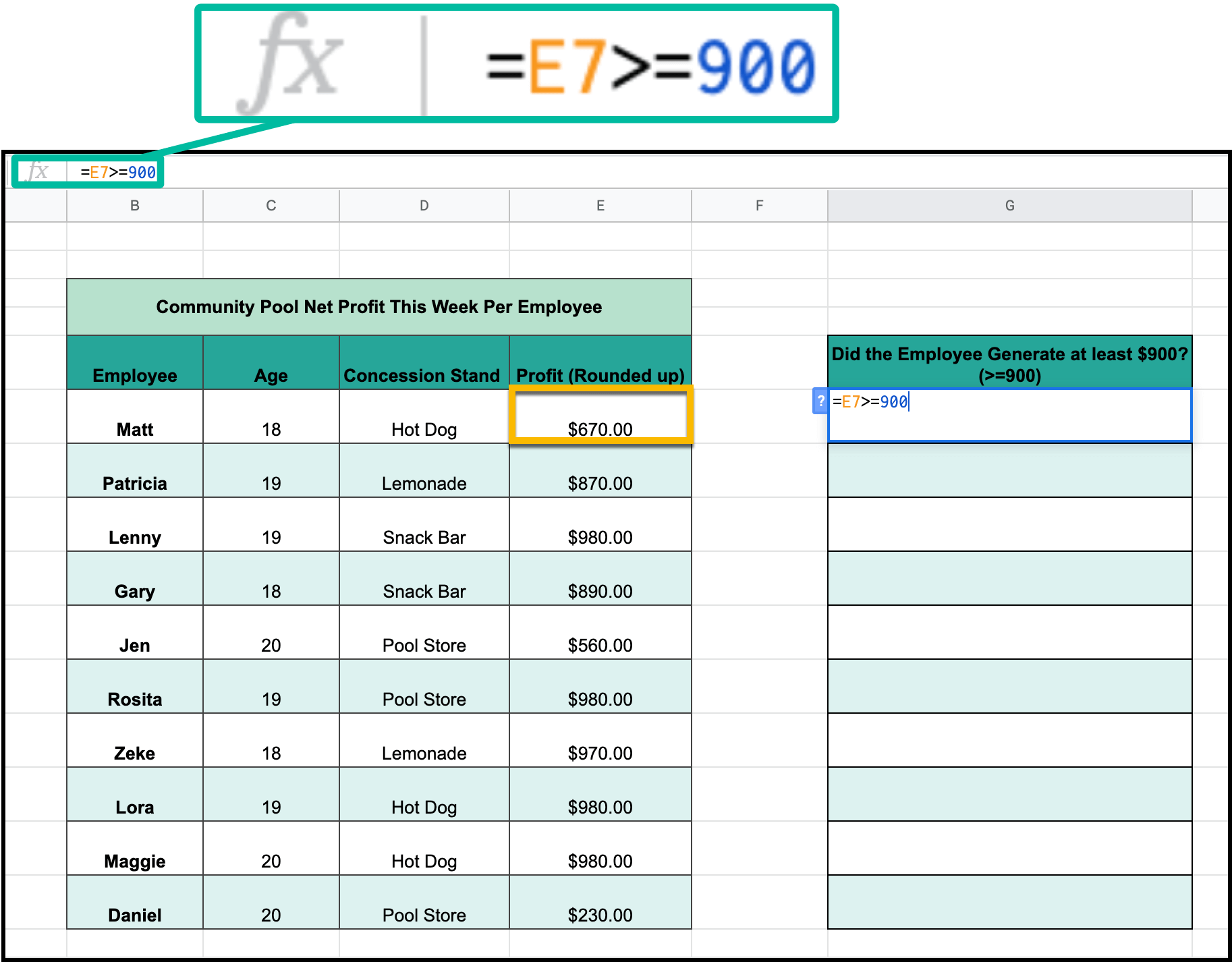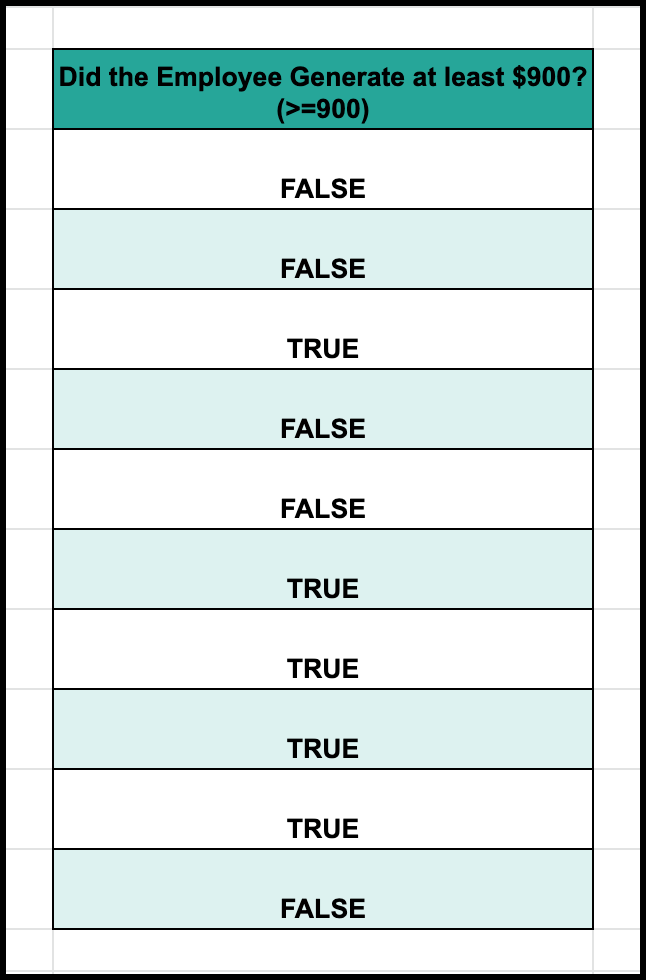# Logical Tests and The Foundation of Conditional Functions in Google SheetsLearning how to run a logical test is the foundation for understanding the more confusing if-then statement functions later on in Sheets. Today, I will show you how to use and understand logical tests in Google Sheets.

Like I said, it is a simple concept, but it is vital to understand it for the more complex conditional functions that you could encounter later on.

This includes formulas such as:

## Conditional StatementsYou will first need to understand the concept of True and False and why it matters. Numerically, you will need to understand how to find each type of numerical condition.

Greater Than: "=Cell>Minimum Value"

Greater Than or Equal to: "=Cell>=Minimum Value"

Less Than: "=Cell<Maximum Value"

Less Than or Equal to: "=Cell<=Maximum Value"

Equals: "=Cell=Target Value"

Does not Equal: "=Cell<>Target Value"

In the basic mode, without any further code, Google Sheets will assume that you want it to find out if the statement is true or false for the specific cell.

For my example, I wanted to find which employees at my fictional pool made me at least \$900. That means that my equation will look like:

=E7>=900

## True or FalseAs you can see the equation is applied to all of the data and comes back with either a statement of true or false.

## Final Thoughts

Understanding this concept of true/false is important, because a lot of the more complex and powerful functions and features run on a fundament concept of If-Then. Another way to think about it is the statement:

"If X is true, then Y will happen."

If you can master the concept of conditional statements and True/False data scanning, then you have the fundamental skills to master the deeper functions and become a Google Sheets wizard!